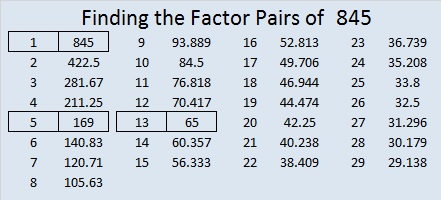# How Often Is 845 the Hypotenuse in a Pythagorean Triple?

Mathematics is a wonderful collection of patterns.

If we look at the patterns of Pythagorean triples, can we predict how often 845 is the hypotenuse of a Pythagorean triple? Yes, we can.

The prime factorization of 845 is 5 × 13². Now 5, 13, 169 each has one primitive triple while 65 has two primitive triples. That’s a total of five primitive triples. Each of those primitives has a multiple with 845 as the hypotenuse.

1. 5’s primitive (3-4-5) times 169 is 507-676-845
2. 13’s primitive (5-12-13) times 65 is 325-780-845
3. 169’s primitive (119-120-169) times 5 is 595-600-845
4. 65’s first primitive (16-63-65) times 13 is 208-819-845
5. 65’s second primitive (33-56-65) times 13 is 429-728-845

Because ALL of its prime factors are primitive Pythagorean triple hypotenuses, 845 will also have some primitives of its own. How many?

Now 325, which is 5² × 13, has five non-primitive triples and two primitive triples, 36-323-325 and 204-253-325 . Since 325 and 845 have the same number of non-primitive triples, and similar looking prime factorizations, is it reasonable to assume that 845 will have two primitive triples as well? Yes, it is!

We can find 845’s primitive Pythagorean triples by looking at the ways to write 845 as the sum of two squares:29² + 2² = 845, 26² + 13² = 845, and 22² + 19² = 845

26 is a multiple of 13 so it will duplicate one of the triples given by one of 845’s factors. The other two equations give us these two primitive Pythagorean triples:

1. 116-837-845, which was calculated from 2(29)(2), 29² – 2², 29² + 2²
2. 123-836-845, which was calculated from 22² – 19², 2(22)(19), 22² + 19²

Thus 845 has a total of 5 + 2 = 7 Pythagorean triples in which it is the hypotenuse!

What if we wanted to know how many times 5² × 13² = 4225 is the hypotenuse of a Pythagorean triple. Could we predict how many times that would be? Yes, we can!

Besides 1 and itself, the factors of 4225 are 5, 13, 25, 65, 169, 325, and 845, all Pythagorean triple hypotenuses. Each of their respective primitive Pythagorean triples has a multiple with 4225 as the hypotenuse:

1. 845 times 5’s primitive
2. 325 times 13’s primitive
3. 169 times 25’s primitive
4. 25 times 169’s primitive
5. 65 times 65’s two primitives
6. 13 times 325’s two primitives
7. 5 times 845’s two primitives

That’s a total of 10 non-primitive Pythagorean triples for 4225.

We know that 4225 will have some primitives of its own. If the pattern holds true, I hypothesize that 4225 will also have two primitives. So I ask, “What sums of two squares equal 4225?”

• 63² + 16² = 4225; 63 and 16 have no common prime factors so this will produce a primitive triple.
• 60² + 25² = 4225; 60 and 25 have 5 as a common factor so this will duplicate one of 4225’s other non-primitives.
• 56² + 33² = 4225; 56 and 33 have no common prime factors so this will produce a primitive triple.
• 52² + 39² = 4225; 52 and 39 have 13 as a common factor so this will duplicate one of 4225’s other non-primitives.

Thus the pattern held true and my hypothesis was correct! 4225 has a total of 10 + 2 = 12 Pythagorean triples! All of this has led me to some conclusions:

If x is a prime number with a remainder of 1 when divided by 4, and a is an integer that is greater than or equal to one, then xᴬ will have one primitive triple while the total number of Pythagorean triples will be A.

If x and y are prime numbers each with a remainder of 1 when divided by 4, and if A and B are integers with B ≥ A and A ≥ 1, then xᴬ × y will have two primitive triples. It appears that the total number of triples xᴬ × yᴮ will have will be A + B + 2Bᴬ. That is my conjecture.

Let’s check my conjecture for the numbers discussed in this post:

• 5¹ and 13¹ each have 1 triple
• 5² and 13² each have 2 triples
• 5² × 13¹ and 5¹ × 13² each have 1 + 2 + 2(2¹) = 7 triples
• 5² × 13² has 2 + 2 + 2(2²) = 12 triples

So far the conjecture appears to ring true. Perhaps I’ll explore it a little more when I write post 2 × 5² × 17¹ later this week.

Now here’s a little more about the number 845:

844, 845, 846, 847, and 848 are the smallest five consecutive numbers whose square roots can be simplified.

845 is palindrome 5A5 in BASE 12 (A is 10 base 10) because 5(12²) + 10(12¹) + 5(12º) = 845

845 is 500 in BASE 13 because 5(13²) = 845

• 845 is a composite number.
• Prime factorization: 845 = 5 × 13 × 13, which can be written 845 = 5 × 13²
• The exponents in the prime factorization are 1 and 2. Adding one to each and multiplying we get (1 + 1)(2 + 1) = 2 × 3  = 6. Therefore 845 has exactly 6 factors.
• Factors of 845: 1, 5, 13, 65, 169, 845
• Factor pairs: 845 = 1 × 845, 5 × 169, or 13 × 65
• Taking the factor pair with the largest square number factor, we get √845 = (√169)(√5) = 13√5 ≈ 29.06888## One thought on “How Often Is 845 the Hypotenuse in a Pythagorean Triple?”

This site uses Akismet to reduce spam. Learn how your comment data is processed.